Latest Banking jobs   »   Quantitative Aptitude Quiz For ESIC- UDC,...

# Quantitative Aptitude Quiz For ESIC- UDC, Steno, MTS Prelims 2022-18th January

Directions (1-5): Study the pie-chart carefully and answer the questions.
Pie-chart given below shows the percentage distribution of students in different class of school ‘RPM’
Note → Ratio of total student of school RPM to school SVM is 2 : 3 and both school has 5 class only i.e. class 6 to class 10
Number of students in 10th class of RPM school is 360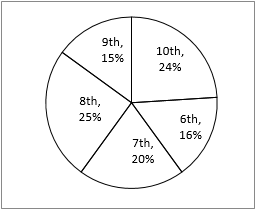Q1. If total student in 6th class in school SVM is 20% of total student then find difference of student in 6th class in both school?
(a) 120
(b) 310
(c) 230
(d) 210
(e)180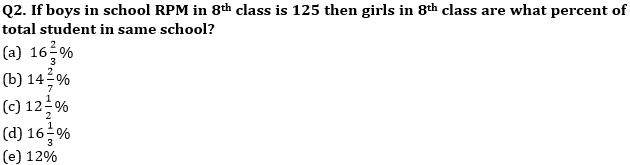Q3. If ratio of students in class 10th of school SVM to that of school RPM is 1 : 2 then students in class 10th in SVM is what percent total student in school?
(a) 9%
(b) 6%
(c) 8%
(d) 4%
(e) 12%

Q4. Find the average no. of students in class 8th and 9th of school SVM if percentage distribution is same as school RPM?
(a) None of these
(b) 425
(c) 400
(d) 250
(e) 450

Q5. If total girls in school RPM is 600 then total boys in school RPM is what percent of total student in SVM?
(a) 40%
(b) 30%
(c) 35%
(d) None of these
(e) 50%

Directions (6-10): Study the passage carefully and answer the questions.
Given below is the data for four countries i.e. India, Pakistan, Iran, France
Price of diesel in Pakistan is Rs50/L which is 37 ½ % less than price of India. Ratio of price of diesel in Pak to Iran is 5 : 2 and average of diesel price including all countries is Rs65/L. Ratio of price of petrol to price of diesel in India is 9 : 8. Price of petrol in India is 260% more them price of petrol in Iran. Average price of petrol in all countries is thrice of price of petrol in Iran.

Q6. What is the difference in sum of price of petrol from all countries & sum of price of diesel from all countries?
(a) Rs 40
(b) Rs 35
(c) None of these
(d) Rs 30
(e) Rs 45

Q7. If price of petrol in India is 50% more than price of petrol in Pak then find difference in price of petrol in Pak and France?
(a) None of these
(b) Rs 45
(c) Rs 65
(d) Rs 60
(e) Rs 75

Q8. What is ratio of price of petrol in Pak and France together to price of diesel in both countries together?
(a) 32 : 27
(b) 37 : 31
(c) 3 : 2
(d) 37 : 32
(e) None of these

Q9. Price of petrol is what percent of price of diesel in Iran?
(a) 25%
(b) 150%
(c) None of these
(d) 75%
(e) 125%

Q10. Difference of price of petrol & diesel in India is what percent of difference in price of petrol & diesel in Pak?
(a) 100%
(b) 50%
(c) None of these
(d) Cannot be determined
(e) 125%

Directions (11–15): Given bar graph shows the number of student passed in Xth class from 6 different school.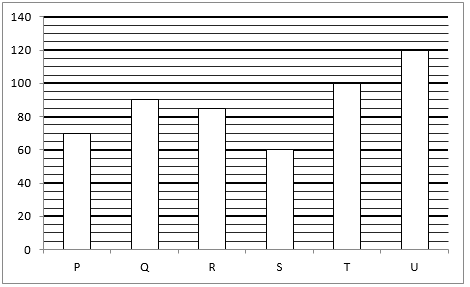Q11. Pass percentage of school S is equal to that of school Q. Find total strength of school Q is what % more than that of school S.
(a) 20%
(b) 40%
(c) 50%
(d) 25%
(e) 60%

Q12. If fail percentage of school P is 65% then, find number of student failed from school P is what percentage of number of student passed from school T.
(a) 30%
(b) 100%
(c) 120%
(d) 130%
(e) 70%

Q13. If ratio between total student who passed to who failed from all school is 7 : 3, then find the total number of failed student from all schools together.
(a) 225
(b) 125
(c) 250
(d) 275
(e) None of these

Q14. Student passed from school P, Q, U and T together is how much more than that of school R and S together.
(a) 220
(b) 250
(c) 190
(d) 220
(e) None of these

Q15. Failed student of school U is 15 more than that of school R. If ratio between total strength of school U to school R is 3 : 2, then find the total number of failed student from both schools together.
(a) 57
(b) 23
(c) 45
(d) 63
(e) 35

Solutions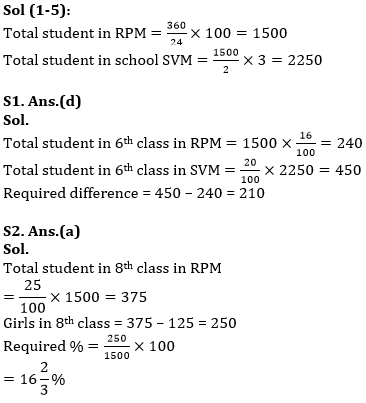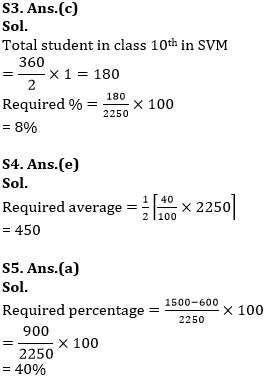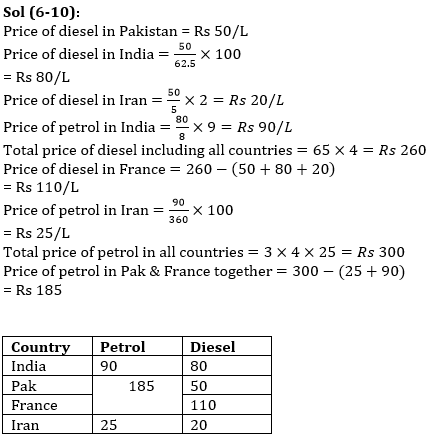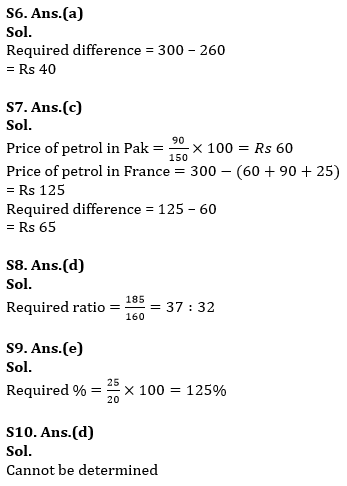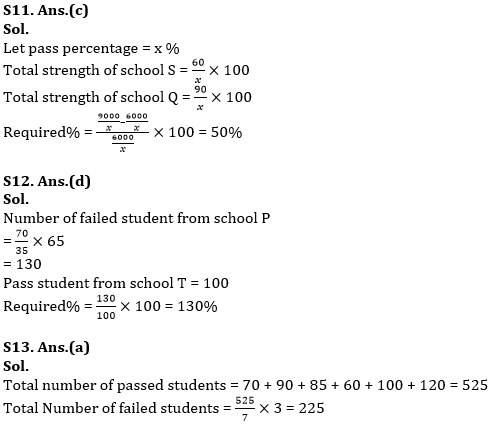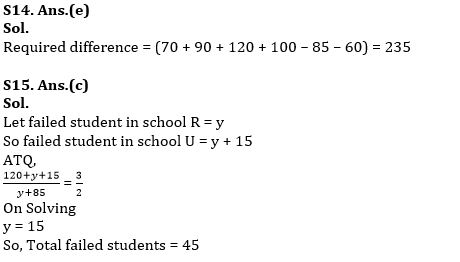#### Congratulations!Union Budget 2023-24: Free PDF